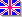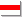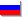### Basic concepts of Riemann geometry

Riemann geometry deals with geometric objects in non-linear space, such as the surface of the sphere etc. First of all let's define some objects in such a space. Let's consider a transformation of coordinates:
yi=yi(x1,...,xn)
If the coordinates of some vector A is transformed as a differential, i.e.
dxi=(dxi/dyk)*dyk  (twin indexes means the sum)
that is Axi=(dxi/dyk)*Ayk for the vector A,
this vector A is defined as the contravariant vector. Otherwise, if the coordinates of vector A is transformed as a components of gradient, i.e.
df/dxi=(dyk/dxi)*(df/dyk)
that is Axi=(dxi/dyk)*Ayk for the vector A,
this vector A is defined as the covariant vector.

Tensor is formally written as the matrix, which components Cij is the multiplied components of some two vectors:
Cij=Ai*Bj
So contravariant tensor Aij with two indexes is such a multiplication of two contravariant vectors, covariant tensor Aij with two indexes is the multiplication of two covariant vectors, mixed tensor with two indexes Aij is the multiplication of covariant and contravariant vectors. Tensor with n contravariant and m covariant indexes Ai1..inj1..jm can be presented as the multiplication of n contravariant and m covariant vectors.

In Riemann geometry Einstein's agreement of twin indexes sum is restricted: the sum can only be obtained from covariant and contravariant indexes. For example:
(A*B)=Ai*Bi
is the scalar multiplication of vectors A and B. Note that coordinates is always contravariant, and differentiation adds to any tensor one covariant index.

The most important mathematical object in Riemann geometry is the metric tensor gij: the distance between two infinitely close points in Riemann space is:
ds2=gijdxidxj
Tensor gij is symmetrical:
gij = gji
The contravariant tensor gij is defined from such an equality:
gikgkj=Eij,
where Eij is the single matrix or Kroneker symbol. Differential of volume is defined as:
dV=(g1/2)*dx1..dxn
where g1/2 is determinant of gij.

Using the tensors gij and gij you can rise and lower indexes. For example:
Ai=gijAj
Aij=gikgjlAkl
Scalar multiplication can be defined in such a way:
(A*B)=AiBjgij

The most fruitful idea of Riemann geometry is a special way of differentiating mathematical objects. The components of vectors or tensors in Riemann space are changed during the parralel translations, because the space is not linear. So the generalized derivative of vector can be written as:
DAi/dxl=dAi/dxl + CiklAk
where Cikl is some set of numbers, which depends on coordinates. This object, as will be shown further, is not a covariant nor a mixed tensor, is usually called coefficient of connexion or connectivity parameter. Differentiang vector Ai by coordinate xj is signed as:
DAi/dxj=Ai;j
A derivative of tensor with two indexes is:
Aik;l=dAik/dxl - CmilAmk - CmklAim
It's evident that derivative defined in such a way derives all the properties of simple algebraic derivative, for example:
(AiBk);l=AiBk;l + Ai;lBk
Let's prove that differential of metric tensor is equal to zero. Differential DAi is vector, so:
DAi=gikDAk, but
Ai=gikAk, and then
DAi=D(gikAk)=gikDAk + AkDgik
Comparing the first and the last statements it's easy to guess that Dgik=0. So metric tensor in Riemann geometry is constant, although it depends on coordinates! To express Cikl through components of metric tensor, let's differentiate it using previously described method and then assume the derivative to zero:
gik;l=dgik/dxl - gmkCmil - gimCmkl = dgik/dxl - Ck,il - Ci,kl = 0
dgik/dxl=Ck,il + Ci,kl where Cikl=gimCm,kl
Finally, after swapping indexes and adding equations with different indexes, we obtain such an expression for Cikl:
Cikl=gim(dgmk/dxl + dgml/dxk - dgkl/dxm)/2
Here is a useful expression for transformations of Cikl:
Ci xkl = Cm ynp(dxi/dym)(dyn/dxk)(dyp/dxl) + (d2ym/dxkdxl)(dxi/dym)
It's evident now that Cikl is not a tensor. Cikl is symmetrical relative to two lower indexes:Cikl = Cilk

Here are some useful expressions for vector operators in Riemann space:

Here g is determinant of gik

Let's define the curvature of Riemann space. We'll consider a parralel translation of some vector Aiover a small contour and find out it's change. Then remember Stock's theorem (see rotor/curl). Finally
dAk=((dCikm/dxl)Ai - (dCikl/dxm)Ai + Cikm(dAi/dxl) - Cikl(dAi/dxm))*dSlm/2
where dSlm is the differential of area of the contour.This can be written as:
dAk=RiklmAidSlm,
where Riklm is curvature tensor:
Riklm=dCikm/dxl - dCikl/dxm + CinlCnkm - CinmCnkl
Here are another useful expression for Riklm:
Ai;k;l - Ai;l;k = AmRmikl
Some properties of the curvature tensor are:

• Riklm=-Rikml
• Riklm + Rimkl + Rilmk = 0
• Rnikl;m + Rnimk;l + Rnilm;k = 0

From curvature tensor with four indexes we can obtain simplified curvature tensor with two indexes:
Rik=Rlilk
Here is another expression for Rik:
Rik=dClik/dxl - dClil/dxk + ClikCmlm - CmilClkm
This tensor is simmetrical:
Rik=Rki.
Scalar curvature of the space is defined as:
R=gijRij.

Here is the Delphi unit Riemann.pas specially designed for analysing the properties of Riemann space. You just have to define metric tensors gij and gij in functions MetricDown and MetricUp and the dimension of the space N. If it's difficult to evaluate the inverse matrixes, you can use unit Matrix.pas

©2002-2003, VeterEnglishБеларускаяРусский
Сайт создан в системе uCoz